## Irrational Number

A number which cannot be expressed as a Fraction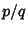for any Integers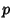and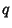. Every Transcendental Number is irrational. Numbers of the form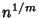are irrational unlessis theth Power of an Integer.

Numbers of the form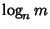, where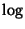is the Logarithm, are irrational ifandare Integers, one of which has a Prime factor which the other lacks.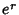is irrational for rational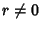. The irrationality of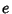was proven by Lambertin 1761; for the general case, see Hardy and Wright (1979, p. 46).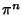is irrational for Positive integral. The irrationality of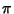was proven by Lambertin 1760; for the general case, see Hardy and Wright (1979, p. 47). Apéry's Constant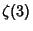(where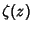is the Riemann Zeta Function) was proved irrational by Apéry (Apéry 1979, van der Poorten 1979).

From Gelfond's Theorem, a number of the form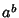is Transcendental (and therefore irrational) ifis Algebraic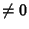, 1 and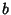is irrational and Algebraic. This establishes the irrationality of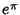(since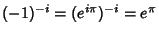),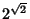, and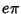. Nesterenko (1996) proved that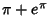is irrational. In fact, he proved that,and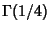are algebraically independent, but it was not previously known thatwas irrational.

Given a Polynomial equation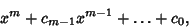(1)

where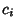are Integers, the roots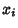are either integral or irrational. If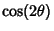is irrational, then so are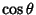,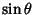, and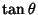.

Irrationality has not yet been established for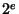,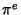,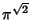, or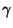(whereis the Euler-Mascheroni Constant).

Quadratic Surds are irrational numbers which have periodic Continued Fractions.

Hurwitz's Irrational Number Theorem gives bounds of the form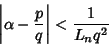(2)

for the best rational approximation possible for an arbitrary irrational number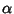, where the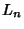are called Lagrange Numbers and get steadily larger for each bad'' set of irrational numbers which is excluded.

The Series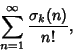(3)

where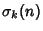is the Divisor Function, is irrational for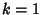and 2. The series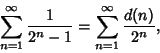(4)

where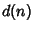is the number of divisors of, is also irrational, as are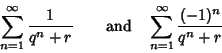(5)

foran Integer other than 0 and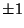, and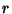a Rational Number other than 0 or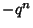(Guy 1994).

See also Algebraic Integer, Algebraic Number, Almost Integer, Dirichlet Function, Ferguson-Forcade Algorithm, Gelfond's Theorem, Hurwitz's Irrational Number Theorem, Near Noble Number, Noble Number, Pythagoras's Theorem, Quadratic Irrational Number, Rational Number, Segre's Theorem, Transcendental Number

ReferencesIrrational Numbers

Apéry, R. Irrationalité de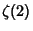et.'' Astérisque 61, 11-13, 1979.

Courant, R. and Robbins, H. Incommensurable Segments, Irrational Numbers, and the Concept of Limit.'' §2.2 in What is Mathematics?: An Elementary Approach to Ideas and Methods, 2nd ed. Oxford, England: Oxford University Press, pp. 58-61, 1996.

Guy, R. K. Some Irrational Series.'' §B14 in Unsolved Problems in Number Theory, 2nd ed. New York: Springer-Verlag, p. 69, 1994.

Hardy, G. H. and Wright, E. M. An Introduction to the Theory of Numbers, 5th ed. Oxford, England: Clarendon Press, 1979.

Manning, H. P. Irrational Numbers and Their Representation by Sequences and Series. New York: Wiley, 1906.

Nesterenko, Yu. Modular Functions and Transcendence Problems.'' C. R. Acad. Sci. Paris Sér. I Math. 322, 909-914, 1996.

Nesterenko, Yu. V. Modular Functions and Transcendence Questions.'' Mat. Sb. 187, 65-96, 1996.

Niven, I. M. Irrational Numbers. New York: Wiley, 1956.

Niven, I. M. Numbers: Rational and Irrational. New York: Random House, 1961.

Pappas, T. Irrational Numbers & the Pythagoras Theorem.'' The Joy of Mathematics. San Carlos, CA: Wide World Publ./Tetra, pp. 98-99, 1989.

van der Poorten, A. A Proof that Euler Missed... Apéry's Proof of the Irrationality of.'' Math. Intel. 1, 196-203, 1979.